SPSS TUTORIALS BASICS ANOVA REGRESSION FACTOR CORRELATION

# SPSS Scatterplots & Fit Lines Tool

Visualizing your data is the single best thing you can do with it. Doing so may take little effort: a single line FREQUENCIES command in SPSS can create many histograms or bar charts in one go.

Sadly, the situation for scatterplots is different: each of them requires a separate command. We therefore built a tool for creating one, many or all scatterplots among a set of variables, optionally with (non)linear fit lines and regression tables.

## Example Data File

We'll use health-costs.sav (partly shown below) throughout this tutorial.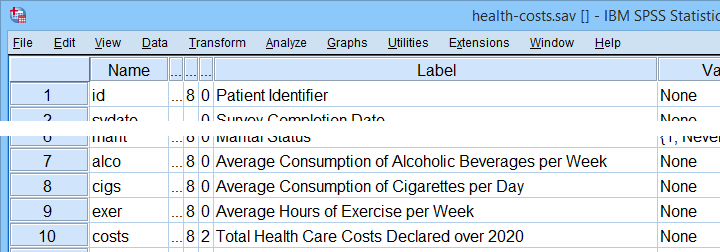We encourage you to download and open this file and replicate the examples we'll present in a minute.

## Prerequisites and Installation

Our tool requires SPSS version 24 or higher. Also, the SPSS Python 3 essentials must be installed (usually the case with recent SPSS versions).

Clicking SPSS_TUTORIALS_SCATTERS.spe downloads our scatterplots tool. You can install it through ExtensionsInstall local extension bundle as shown below.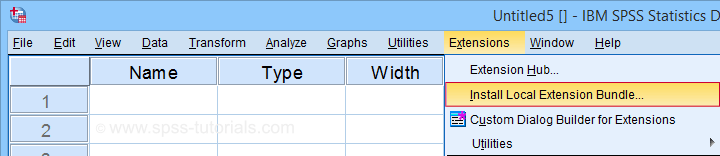In the dialog that opens, navigate to the downloaded .spe file and install it. SPSS will then confirm that the extension was successfully installed under GraphsSPSS tutorials - Create All Scatterplots

## Example I - Create All Unique Scatterplots

Let's now inspect all unique scatterplots among health costs, alcohol and cigarette consumption and exercise. We'll navigate to GraphsSPSS tutorials - Create All Scatterplots and fill out the dialog as shown below.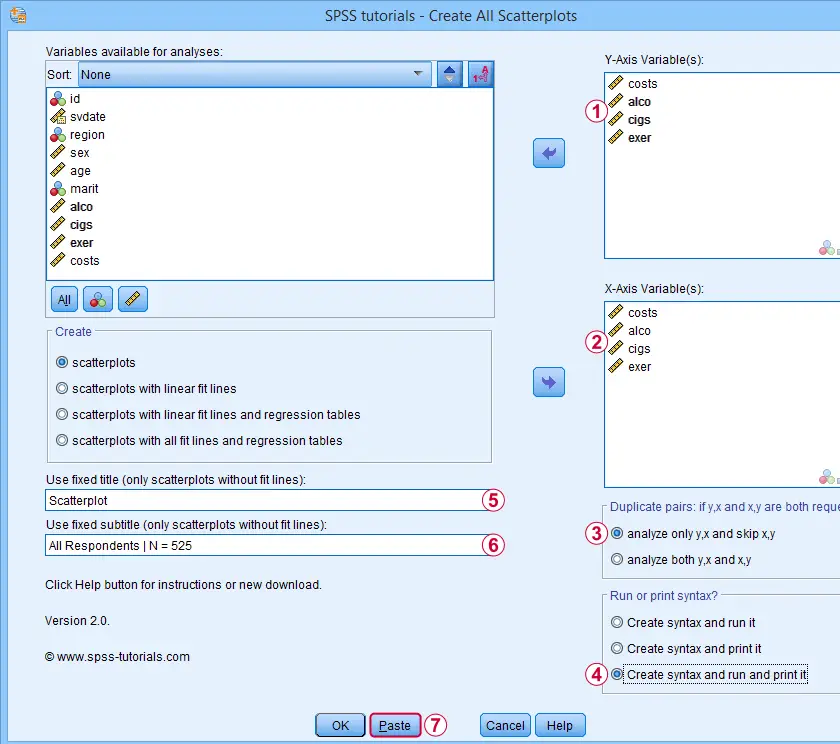We enter all relevant variables as y-axis variables. We recommend you always first enter the dependent variable (if any).We enter these same variables as x-axis variables.This combination of y-axis and x-axis variables results in duplicate chart. For instance, costs by alco is similar alco by costs transposed. Such duplicates are skipped if “analyze only y,x and skip x,y” is selected.Besides creating scatterplots, we'll also take a quick look at the SPSS syntax that's generated.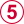If no title is entered, our tool applies automatic titles. For this example, the automatic titles were rather lengthy. We therefore override them with a fixed title (“Scatterplot”) for all charts. The only way to have no titles at all is suppressing them with a chart template.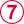Clicking results in the syntax below. Let's run it.

## SPSS Scatterplots Tool - Syntax I

*Create all unique scatterplots among costs, alco, cigs and exer.

SPSS TUTORIALS SCATTERS YVARS=costs alco cigs exer XVARS=costs alco cigs exer
/OPTIONS ANALYSIS=SCATTERS ACTION=BOTH TITLE="Scatterplot" SUBTITLE="All Respondents | N = 525".

## Results

First off, note that the GRAPH commands that were run by our tool have also been printed in the output window (shown below). You could copy, paste, edit and run these on any SPSS installation, even if it doesn't have our tool installed.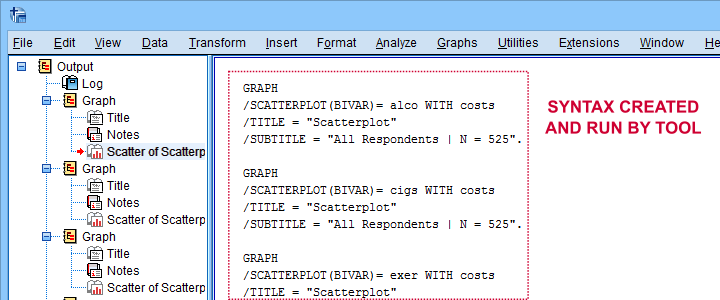Beneath this syntax, we find all 6 unique scatterplots. Most of them show substantive correlations and all of them look plausible. However, do note that some plots -especially the first one- hint at some curvilinearity. We'll thoroughly investigate this in our second example.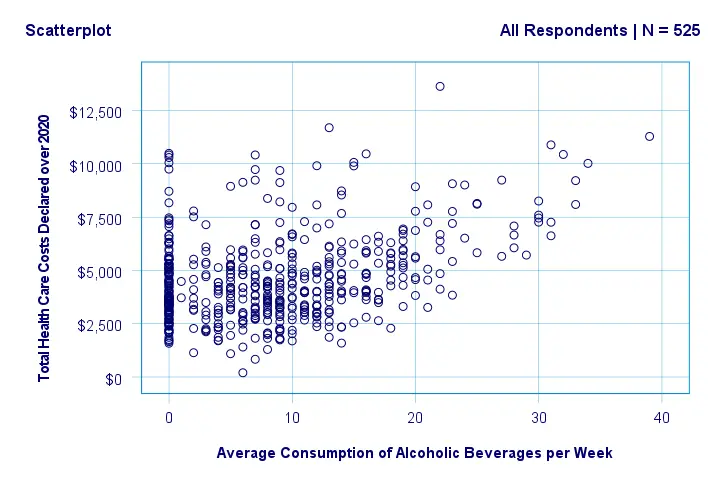In any case, we feel that a quick look at such scatterplots should always precede an SPSS correlation analysis.

## Example II - Linearity Checks for Predictors

I'd now like to run a multiple regression analysis for predicting health costs from several predictors. But before doing so, let's see if each predictor relates linearly to our dependent variable. Again, we navigate to GraphsSPSS tutorials - Create All Scatterplots and fill out the dialog as shown below.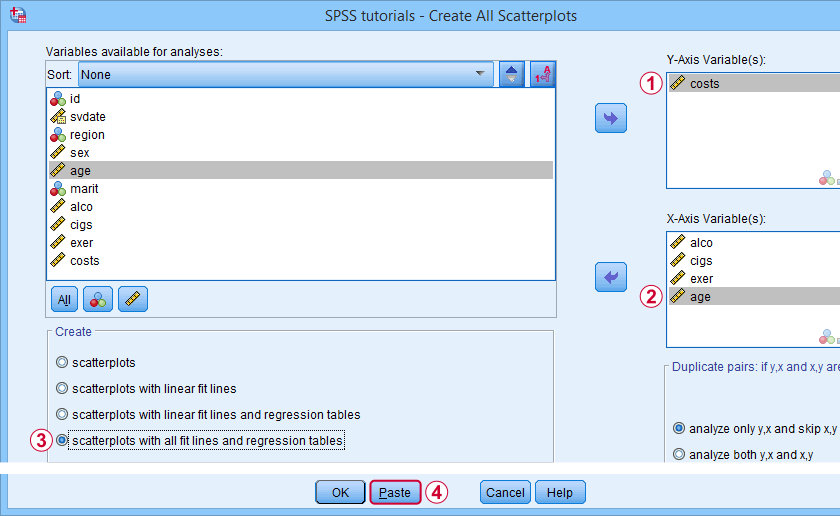Our dependent variable is our y-axis variable.All independent variables are x-axis variables.We'll create scatterplots with all fit lines and regression tables.We'll run the syntax below after clicking the button.

## SPSS Scatterplots Tool - Syntax II

*Fit all possible curves for 4 predictors onto single dependent variable.

SPSS TUTORIALS SCATTERS YVARS=costs XVARS=alco cigs exer age
/OPTIONS ANALYSIS=FITALLTABLES ACTION=RUN.

Note that running this syntax triggers some warnings about zero values in some variables. These can safely be ignored for these examples.

## Results

In our first scatterplot with regression lines, some curves deviate substantially from linearity as shown below.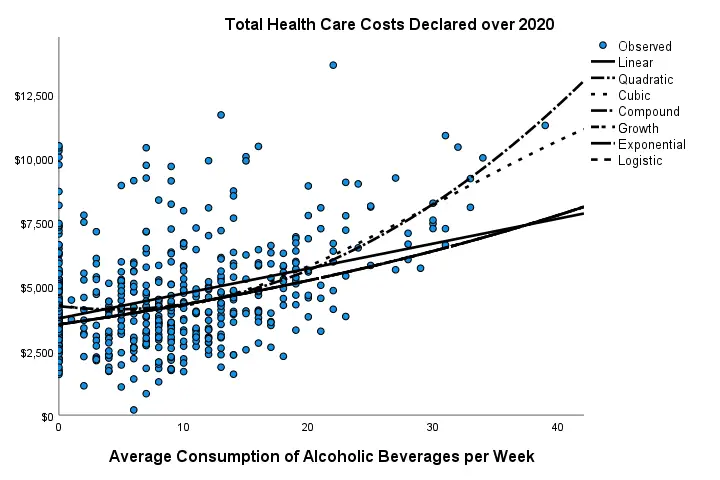Sadly, this chart's legend doesn't quite help to identify which curve visualizes which transformation function. So let's look at the regression table shown below.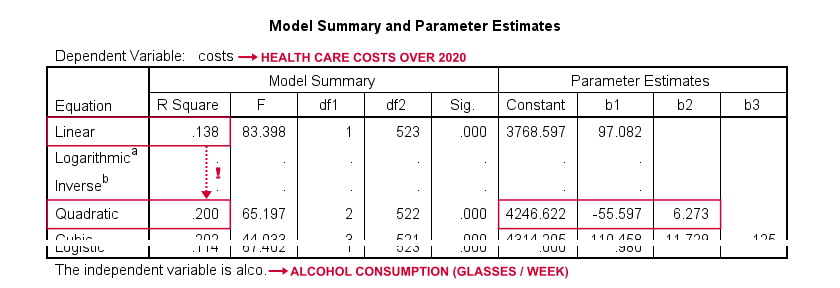Very interestingly, r-square skyrockets from 0.138 to 0.200 when we add the squared predictor to our model. The b-coefficients tell us that the regression equation for this model is Costs’ = 4,246.22 - 55.597 * alco + 6.273 * alco2 Unfortunately, this table doesn't include significance levels or confidence intervals for these b-coefficients. However, these are easily obtained from a regression analysis after adding the squared predictor to our data. The syntax below does just that.

*Compute squared alcohol consumption.

compute alco2 = alco**2.

*Multiple regression for costs on squared and non squared alcohol consumption.

regression
/statistics r coeff ci(95)
/dependent costs
/method enter alco alco2.

## Result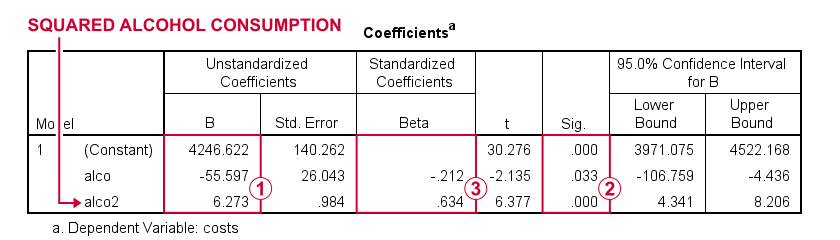First note that we replicated the exact b-coefficients we saw earlier.Surprisingly, our squared predictor is more statistically significant than its original, non squared counterpart.The beta coefficients suggest that the relative strength of the squared predictor is roughly 3 times that of the original predictor.

In short, these results suggest substantial non linearity for at least one predictor. Interestingly, this is not detected by using the standard linearity check: inspecting a scatterplot of standardized residuals versus predicted values after running multiple regression.

But anyway, I just wanted to share the tool I built for these analyses and illustrate it with some typical examples. Hope you found it helpful!

If you've any feedback, we always appreciate if you throw us a comment below.

# Tell us what you think!

*Required field. Your comment will show up after approval from a moderator.

# THIS TUTORIAL HAS 3 COMMENTS:

•### By Jon Peck on November 30th, 2017

In the same spirit as this extension, I would like to point out the STATS REGRESS PLOT (Graphs > Regression ariable Plots) extension that is installed with the Python Essentials or can be installed from the Utilities or Extensions menu.

This was designed to help in screening candidate regressors, including nonlinear transformations, in a regression model. It plots one variable against each of a set of variables in a compact format. The type of plot chosen is based on each variable's measurement level, and there are a number of choices as well as fit line options.

•### By Jon Peck on April 11th, 2021

This is a lot like the STATS REGR PLOTS extension command available from the Extension Hub.

•### By Ruben Geert van den Berg on April 12th, 2021

Hi Jon!

That's right. I tried the STATS REGR PLOTS extension for a while before I created this tool but I preferred some things a bit differently:

-STATS REGR PLOTS didn't allow me to exclude "duplicate pairs": entering v1 and v2 as both x-axis and y-variables always results in 4 plots even if I need only 1
-STATS REGR PLOTS forces me to set all measurement levels to scale
-STATS REGR PLOTS has fewer curve functions than CURVEFIT and
-STATS REGR PLOTS sometimes doesn't show a legend/regression table for different curve functions.

Those are some reasons for creating this tool after trying STATS REGR PLOTS. IIRC, I also experienced some issues with the TO and ALL keywords and incorrect variable casing. IMHO, the user should be able to use any casing he likes for variable names because that goes for basically all native syntax too.

Reversely, STATS REGR PLOTS incorporates a grouping variable which my tool doesn't. However, when comparing groups I usually create only 1 or 2 scatterplots which is doable "manually" via GRAPH or GPL.

Hope that clarifies!

Ruben